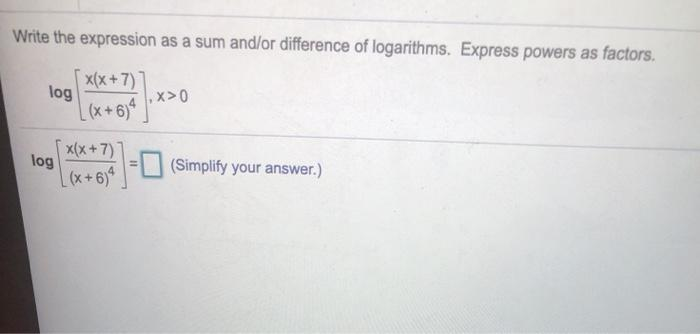### Create an Account

Already have account?

### Forgot Your Password ?

Home / Questions / Write the expression as a sum and/or difference of logarithms. Express powers as factors. ...

# Write the expression as a sum and/or difference of logarithms. Express powers as factors. 5 x(x+7)] log ,X>0 [(x +634) x(x + 7) (Simplify your answer.) [(x + 6)] log

Write the expression as a sum and/or difference of logarithms. Express powers as factors. 5 x(x+7)] log ,X>0 [(x +634) x(x + 7) (Simplify your answer.) [(x + 6)] logApr 15 2021 View more View Less

#### Answer (Solved)Subscribe To Get Solution